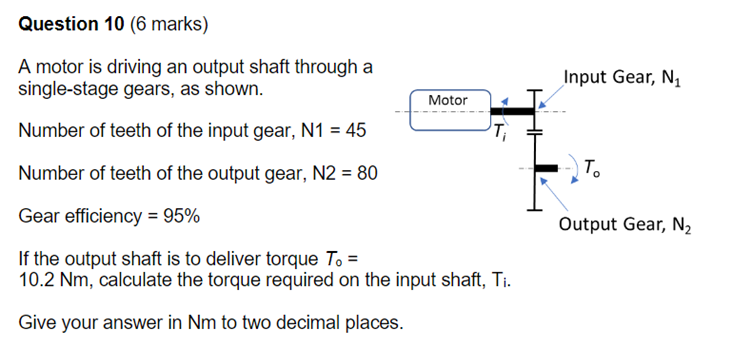# Question Answer is 6.04Nm. How do i get this answer please ? Question 10 (6 marks) A motor is driving an output shaft through a single-stage gears, as shown. Motor Number of teeth of the input gear, N1 = 45 Number of teeth of the output gear, N2 = 80 Gear efficiency = 95% If the output shaft is to deliver torque To = 10.2 Nm, calculate the torque required on the input shaft, Ti. Give your answer in Nm to two decimal places. Input Gear, N₁ To Output Gear, N₂8PMDIH The Asker · Mechanical Engineering